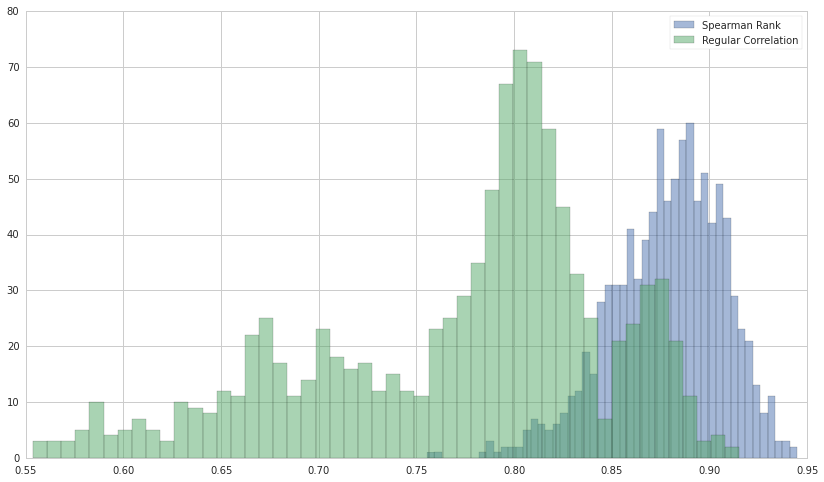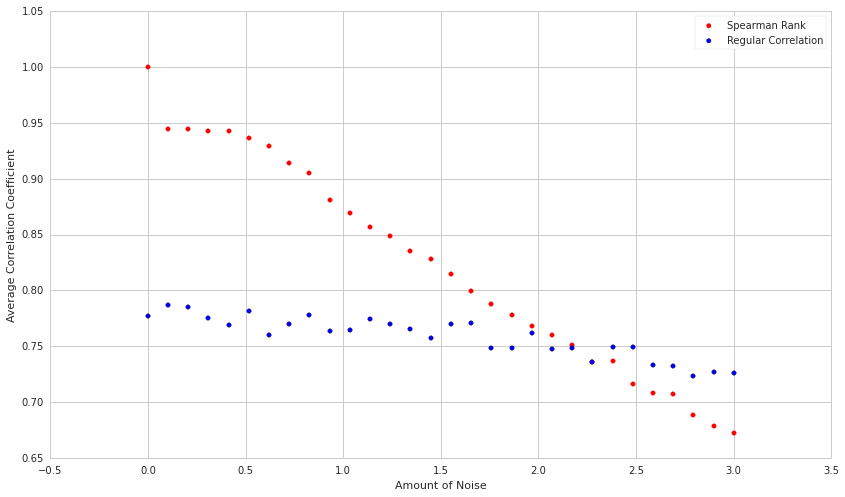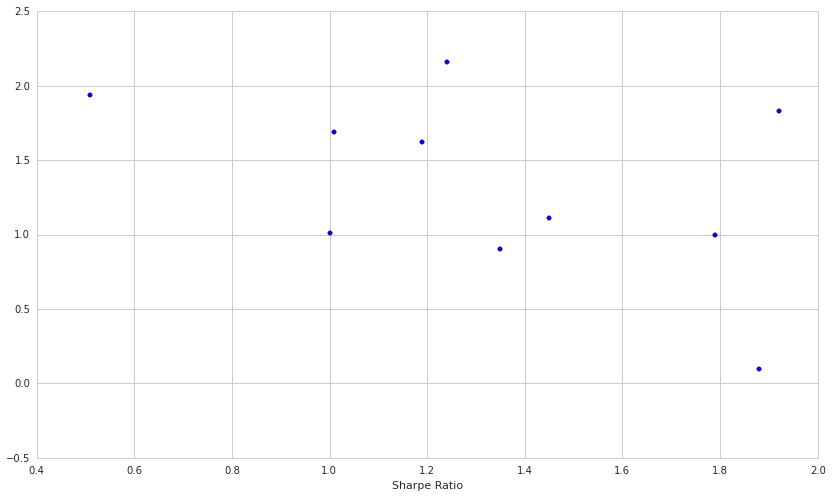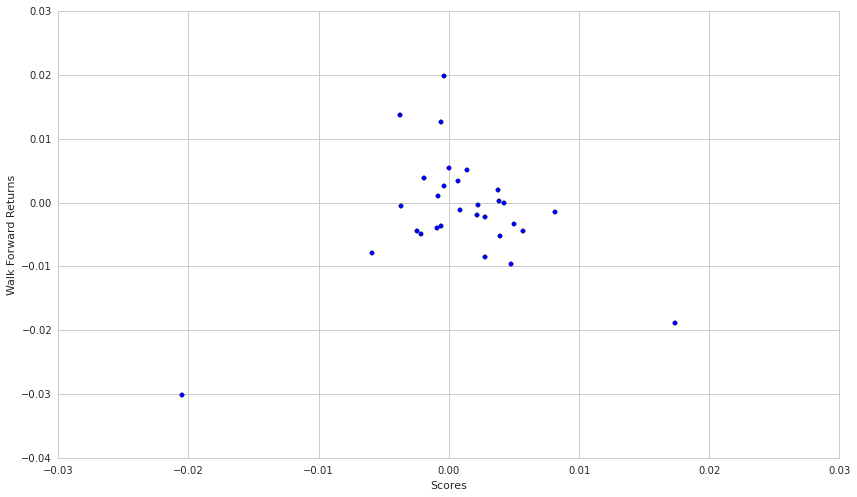Notebook

# Measuring monotonic relationships¶

By Evgenia "Jenny" Nitishinskaya and Delaney Granizo-Mackenzie with example algorithms by David Edwards

Reference: DeFusco, Richard A. "Tests Concerning Correlation: The Spearman Rank Correlation Coefficient." Quantitative Investment Analysis. Hoboken, NJ: Wiley, 2007

Part of the Quantopian Lecture Series:

The Spearman Rank Correlation Coefficient allows us to determine whether or not two data series move together; that is, when one increases (decreases) the other also increases (decreases). This is more general than a linear relationship; for instance, $y = e^x$ is a monotonic function, but not a linear one. Therefore, in computing it we compare not the raw data but the ranks of the data.

This is useful when your data sets may be in different units, and therefore not linearly related (for example, the price of a square plot of land and its side length, since the price is more likely to be linear in the area). It's also suitable for data sets which not satisfy the assumptions that other tests require, such as the observations being normally distributed as would be necessary for a t-test.

In :
import numpy as np
import scipy.stats as stats
import matplotlib.pyplot as plt
import math

In :
# Example of ranking data
l = [10, 9, 5, 7, 5]
print 'Raw data: ', l
print 'Ranking: ', list(stats.rankdata(l, method='average'))

Raw data:  [10, 9, 5, 7, 5]
Ranking:  [5.0, 4.0, 1.5, 3.0, 1.5]


## Spearman Rank Correlation¶

### Intuition¶

The intution is now that instead of looking at the relationship between the two variables, we look at the relationship between the ranks. This is robust to outliers and the scale of the data.

### Definition¶

The argument method='average' indicates that when we have a tie, we average the ranks that the numbers would occupy. For example, the two 5's above, which would take up ranks 1 and 2, each get assigned a rank of $1.5$.

To compute the Spearman rank correlation for two data sets $X$ and $Y$, each of size $n$, we use the formula $$r_S = 1 - \frac{6 \sum_{i=1}^n d_i^2}{n(n^2 - 1)}$$

where $d_i$ is the difference between the ranks of the $i$th pair of observations, $X_i - Y_i$.

The result will always be between $-1$ and $1$. A positive value indicates a positive relationship between the variables, while a negative value indicates an inverse relationship. A value of 0 implies the absense of any monotonic relationship. This does not mean that there is no relationship; for instance, if $Y$ is equal to $X$ with a delay of 2, they are related simply and precisely, but their $r_S$ can be close to zero:

## Experiment¶

Let's see what happens if we draw $X$ from a poisson distribution (non-normal), and then set $Y = e^X + \epsilon$ where $\epsilon$ is drawn from another poisson distribution. We'll take the spearman rank and the correlation coefficient on this data and then run the entire experiment many times. Because $e^X$ produces many values that are far away from the rest, we can this of this as modeling 'outliers' in our data. Spearman rank compresses the outliers and does better at measuring correlation. Normal correlation is confused by the outliers and on average will measure less of a relationship than is actually there.

In :
## Let's see an example of this
n = 100

def compare_correlation_and_spearman_rank(n, noise):
X = np.random.poisson(size=n)
Y = np.exp(X) + noise * np.random.normal(size=n)

Xrank = stats.rankdata(X, method='average')
# n-2 is the second to last element
Yrank = stats.rankdata(Y, method='average')

diffs = Xrank - Yrank # order doesn't matter since we'll be squaring these values
r_s = 1 - 6*sum(diffs*diffs)/(n*(n**2 - 1))
c_c = np.corrcoef(X, Y)[0,1]

return r_s, c_c

experiments = 1000
spearman_dist = np.ndarray(experiments)
correlation_dist = np.ndarray(experiments)
for i in range(experiments):
r_s, c_c = compare_correlation_and_spearman_rank(n, 1.0)
spearman_dist[i] = r_s
correlation_dist[i] = c_c

print 'Spearman Rank Coefficient: ' + str(np.mean(spearman_dist))
# Compare to the regular correlation coefficient
print 'Correlation coefficient: ' + str(np.mean(correlation_dist))

Spearman Rank Coefficient: 0.878350309031
Correlation coefficient: 0.774340023872


Let's take a look at the distribution of measured correlation coefficients and compare the spearman with the regular metric.

In :
plt.hist(spearman_dist, bins=50, alpha=0.5)
plt.hist(correlation_dist, bins=50, alpha=0.5)
plt.legend(['Spearman Rank', 'Regular Correlation'])
plt.xlabel

Out:
<function matplotlib.pyplot.xlabel>Now let's see how the Spearman rank and Regular coefficients cope when we add more noise to the situation.

In :
n = 100
noises = np.linspace(0, 3, 30)
experiments = 100
spearman = np.ndarray(len(noises))
correlation = np.ndarray(len(noises))

for i in range(len(noises)):
# Run many experiments for each noise setting
rank_coef = 0.0
corr_coef = 0.0
noise = noises[i]
for j in range(experiments):
r_s, c_c = compare_correlation_and_spearman_rank(n, noise)
rank_coef += r_s
corr_coef += c_c
spearman[i] = rank_coef/experiments
correlation[i] = corr_coef/experiments

plt.scatter(noises, spearman, color='r')
plt.scatter(noises, correlation)
plt.legend(['Spearman Rank', 'Regular Correlation'])
plt.xlabel('Amount of Noise')
plt.ylabel('Average Correlation Coefficient')

Out:
<matplotlib.text.Text at 0x7f05c213d5d0>We can see that the Spearman rank correlation copes with the non-linear relationship much better at most levels of noise. Interestingly, at very high levels, it seems to do worse than regular correlation.

## Delay in correlation¶

Of you might have the case that one process affects another, but after a time lag. Now let's see what happens if we add the delay.

In :
n = 100

X = np.random.rand(n)
Xrank = stats.rankdata(X, method='average')
# n-2 is the second to last element
Yrank = stats.rankdata([1,1] + list(X[:(n-2)]), method='average')

diffs = Xrank - Yrank # order doesn't matter since we'll be squaring these values
r_s = 1 - 6*sum(diffs*diffs)/(n*(n**2 - 1))
print r_s

0.148649864986


Sure enough, the relationship is not detected. It is important when using both regular and spearman correlation to check for lagged relationships by offsetting your data and testing for different offset values.

## Built-In Function¶

We can also use the spearmanr function in the scipy.stats library:

In :
# Generate two random data sets
np.random.seed(161)
X = np.random.rand(10)
Y = np.random.rand(10)

r_s = stats.spearmanr(X, Y)
print 'Spearman Rank Coefficient: ', r_s
print 'p-value: ', r_s

Spearman Rank Coefficient:  0.236363636364
p-value:  0.510885317515


We now have ourselves an $r_S$, but how do we interpret it? It's positive, so we know that the variables are not anticorrelated. It's not very large, so we know they aren't perfectly positively correlated, but it's hard to say from a glance just how significant the correlation is. Luckily, spearmanr also computes the p-value for this coefficient and sample size for us. We can see that the p-value here is above 0.05; therefore, we cannot claim that $X$ and $Y$ are correlated.

## Real World Example: Mutual Fund Expense Ratio¶

Now that we've seen how Spearman rank correlation works, we'll quickly go through the process again with some real data. For instance, we may wonder whether the expense ratio of a mutual fund is indicative of its three-year Sharpe ratio. That is, does spending more money on administration, management, etc. lower the risk or increase the returns? Quantopian does not currently support mutual funds, so we will pull the data from Yahoo Finance.

In :
# Copying data for DXHLX, UGPIX, ALQIX, IIRFX, TMSCX, FHKCX, MCDFX, NRICX, PINHX, ANGLX
expense = [1.35, 1.79, 1.45, 1.88, 1., 1.01, 1.19, 1.92, 0.51, 1.24]
sharpe = [0.9, 1., 1.11, 0.1, 1.01, 1.69, 1.62, 1.83, 1.94, 2.16]

plt.scatter(expense, sharpe)
plt.xlabel('Expense Ratio')
plt.xlabel('Sharpe Ratio')

r_S = stats.spearmanr(expense, sharpe)
print 'Spearman Rank Coefficient: ', r_S
print 'p-value: ', r_S

Spearman Rank Coefficient:  -0.369696969697
p-value:  0.293050075293It looks as though the expense ratio and Sharpe ratio may in fact be anticorrelated. But, we don't consider any of this meaningful as the p-value is above 0.05.

## Real World Use Case: Evaluating a Ranking Model¶

Let's say that we have some way of ranking securities and that we'd like to test how well our ranking performs in practice. In this case our model just takes the mean daily return for the last month and ranks the stocks by that metric.

We hypothesize that this will be predictive of the mean returns over the next month. To test this we score the stocks based on a lookback window, then take the spearman rank correlation of the score and the mean returns over the walk forward month.

In :
symbol_list = ['A', 'AA', 'AAC', 'AAL', 'AAMC', 'AAME', 'AAN', 'AAOI', 'AAON', 'AAP', 'AAPL', 'AAT', 'AAU', 'AAV', 'AAVL', 'AAWW', 'AB', 'ABAC', 'ABAX', 'ABB', 'ABBV', 'ABC', 'ABCB', 'ABCD', 'ABCO', 'ABCW', 'ABDC', 'ABEV', 'ABG', 'ABGB']

# Get the returns over the lookback window
start = '2014-12-01'
end = '2015-01-01'
historical_returns = get_pricing(symbol_list, fields='price', start_date=start, end_date=end).pct_change()[1:]

# Compute our stock score
scores = np.mean(historical_returns)
print 'Our Scores\n'
print scores
print '\n'

start = '2015-01-01'
end = '2015-02-01'
walk_forward_returns = get_pricing(symbol_list, fields='price', start_date=start, end_date=end).pct_change()[1:]
walk_forward_returns = np.mean(walk_forward_returns)
print 'The Walk Forward Returns\n'
print walk_forward_returns
print '\n'

plt.scatter(scores, walk_forward_returns)
plt.xlabel('Scores')
plt.ylabel('Walk Forward Returns')

r_s = stats.spearmanr(scores, walk_forward_returns)
print 'Correlation Coefficient: ' + str(r_s)
print 'p-value: ' + str(r_s)

Our Scores

Equity(24757 [A])      -0.000624
Equity(2 [AA])         -0.003646
Equity(47842 [AAC])     0.002745
Equity(45971 [AAL])     0.005677
Equity(45415 [AAMC])   -0.020459
Equity(21 [AAME])       0.002207
Equity(523 [AAN])       0.003765
Equity(45503 [AAOI])    0.004758
Equity(9681 [AAON])     0.004228
Equity(23175 [AAP])     0.003887
Equity(24 [AAPL])      -0.001870
Equity(40707 [AAT])     0.001433
Equity(27922 [AAU])    -0.000576
Equity(27884 [AAV])     0.003966
Equity(47422 [AAVL])    0.017364
Equity(28378 [AAWW])    0.004993
Equity(66 [AB])        -0.000934
Equity(39927 [ABAC])   -0.005923
Equity(31 [ABAX])       0.000679
Equity(22574 [ABB])    -0.002108
Equity(43694 [ABBV])   -0.002467
Equity(22954 [ABC])    -0.000335
Equity(11232 [ABCB])    0.002142
Equity(39052 [ABCD])   -0.000333
Equity(23176 [ABCO])    0.008131
Equity(47935 [ABCW])    0.002776
Equity(46877 [ABDC])   -0.000825
Equity(45840 [ABEV])    0.000035
Equity(24761 [ABG])     0.000830
Equity(45676 [ABGB])   -0.003712
dtype: float64

The Walk Forward Returns

Equity(24757 [A])      -0.003616
Equity(2 [AA])         -0.000464
Equity(47842 [AAC])    -0.008585
Equity(45971 [AAL])    -0.004467
Equity(45415 [AAMC])   -0.030225
Equity(21 [AAME])      -0.000321
Equity(523 [AAN])       0.001977
Equity(45503 [AAOI])   -0.009537
Equity(9681 [AAON])    -0.000094
Equity(23175 [AAP])     0.000246
Equity(24 [AAPL])       0.003940
Equity(40707 [AAT])     0.005084
Equity(27922 [AAU])     0.012719
Equity(27884 [AAV])    -0.005286
Equity(47422 [AAVL])   -0.018883
Equity(28378 [AAWW])   -0.003406
Equity(66 [AB])        -0.003890
Equity(39927 [ABAC])   -0.007934
Equity(31 [ABAX])       0.003403
Equity(22574 [ABB])    -0.004947
Equity(43694 [ABBV])   -0.004363
Equity(22954 [ABC])     0.002655
Equity(11232 [ABCB])   -0.001951
Equity(39052 [ABCD])    0.019828
Equity(23176 [ABCO])   -0.001423
Equity(47935 [ABCW])   -0.002212
Equity(46877 [ABDC])    0.001030
Equity(45840 [ABEV])    0.005386
Equity(24761 [ABG])    -0.001088
Equity(45676 [ABGB])    0.013701
dtype: float64

Correlation Coefficient: -0.159955506118
p-value: 0.398478813425The p-value indicates that our hypothesis is false and we accept the null hypothesis that our ranking was no better than random. This is a really good check of any ranking system one devises for constructing a long-short equity portfolio.